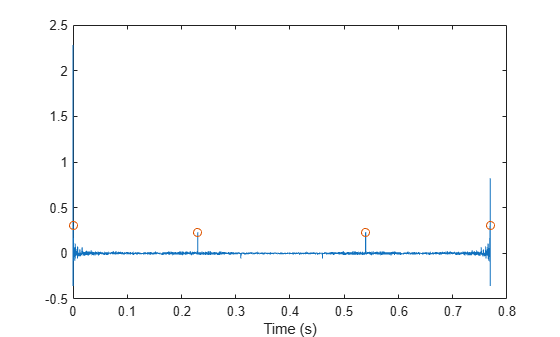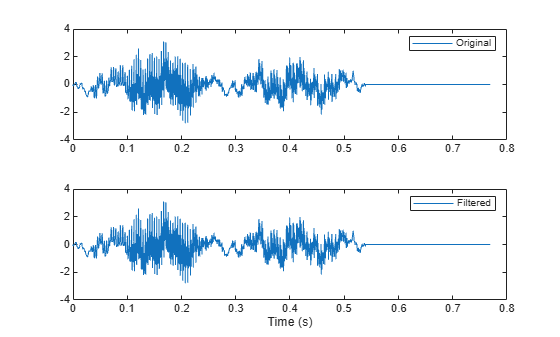rceps

Real cepstrum and minimum-phase reconstruction

Description

example

[y,ym] = rceps(x) returns both the real cepstrum y and a minimum phase reconstructed version ym of the input sequence.

Examples

collapse all

A speech recording includes an echo caused by reflection off a wall. Use the real cepstrum to filter it out.

In the recording, a person says the word MATLAB®. Load the data and the sample rate, ${F}_{s}=7418\phantom{\rule{0.2777777777777778em}{0ex}}Hz$.

% To hear, type soundsc(mtlb,Fs)

Model the echo by adding to the recording a copy of the signal delayed by $\Delta$ samples and attenuated by a known factor $\alpha$: $y\left(n\right)=x\left(n\right)+\alpha x\left(n-\Delta \right)$. Specify a time lag of 0.23 s and an attenuation factor of 0.5.

timelag = 0.23;
delta = round(Fs*timelag);
alpha = 0.5;

orig = [mtlb;zeros(delta,1)];
echo = [zeros(delta,1);mtlb]*alpha;

mtEcho = orig + echo;

Plot the original, the echo, and the resulting signal.

t = (0:length(mtEcho)-1)/Fs;

subplot(2,1,1)
plot(t,[orig echo])
legend('Original','Echo')

subplot(2,1,2)
plot(t,mtEcho)
legend('Total')
xlabel('Time (s)')% To hear, type soundsc(mtEcho,Fs)

Compute the real cepstrum of the signal. Plot the cepstrum and annotate its maxima. The cepstrum has a sharp peak at the time at which the echo starts to arrive.

c = rceps(mtEcho);

[px,locs] = findpeaks(c,'Threshold',0.2,'MinPeakDistance',0.2);

clf
plot(t,c,t(locs),px,'o')
xlabel('Time (s)')Cancel the echo by filtering the signal through an IIR system whose output, $w$, obeys $w\left(n\right)+\alpha w\left(n-\Delta \right)=y\left(n\right)$. Plot the filtered signal and compare it to the original.

dl = locs(2)-1;

mtNew = filter(1,[1 zeros(1,dl-1) alpha],mtEcho);

subplot(2,1,1)
plot(t,orig)
legend('Original')

subplot(2,1,2)
plot(t,mtNew)
legend('Filtered')
xlabel('Time (s)')% To hear, type soundsc(mtNew,Fs)

Input Arguments

collapse all

Input signal, specified as a real vector.

Output Arguments

collapse all

Real cepstrum, returned as a vector.

Minimum phase real cepstrum, returned as a vector.

Algorithms

The real cepstrum is the inverse Fourier transform of the real logarithm of the magnitude of the Fourier transform of a sequence.

Note

rceps only works on real data.

rceps is an implementation of algorithm 7.2 in , that is,

y = real(ifft(log(abs(fft(x)))));

Appropriate windowing in the cepstral domain forms the reconstructed minimum-phase signal:

w = [1;2*ones(n/2-1,1);ones(1-rem(n,2),1);zeros(n/2-1,1)];
ym = real(ifft(exp(fft(w.*y))));

 Oppenheim, Alan V., and Ronald W. Schafer. Digital Signal Processing, Englewood Cliffs, NJ, Prentice-Hall, 1975.

 Programs for Digital Signal Processing, IEEE Press, New York, 1979.